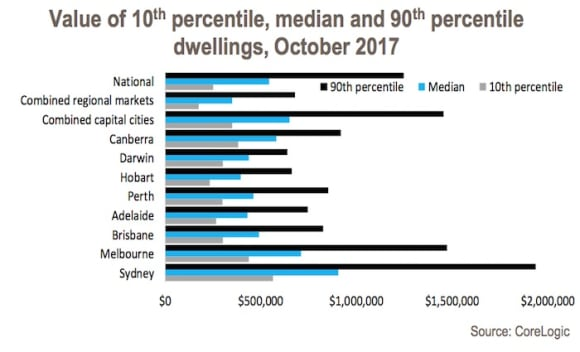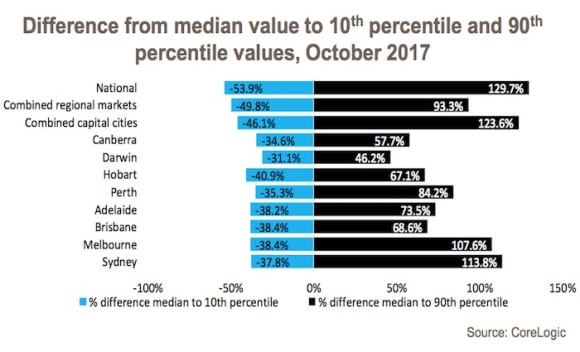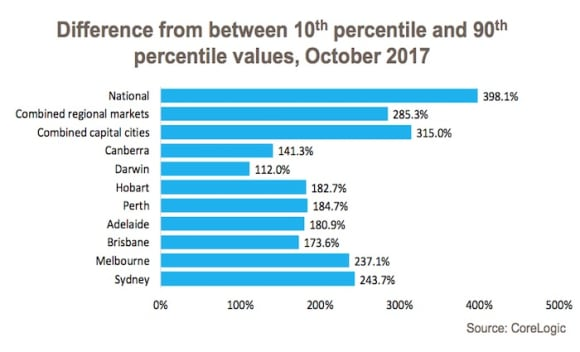# What is the spread in the cost of housing? Cameron KusherTypically, when analyzing housing markets, the median (the middle value) is the most popular method.

By definition the median provides an idea of what a typical property is either selling for or valued at.

By looking at different valuation percentiles, it delivers a much better idea of the range of housing costs across a geography.

(Note: The 10th percentile value represents the lower end of a housing market while the 90th percentile indicates the most expensive 10% of properties).Across Australia’s combined capital cities, the median dwelling value is \$650,930 while the 10th percentile value is \$350,723 and the 90th percentile value is \$1,455,490.

The difference between the median and the 10th percentile value is -46.1% while the difference from the median to the 90th percentile is 123.6% which results in a range between the 10th and 90th percentile values of 315.0%.

(Note: that the broader this range the more diverse the cost of housing and the narrower the less diversity there is).Let’s take a look across the capital cities.

Sydney – the 10th percentile value is \$563,459 which is -37.8% lower than the median of \$905,917. The 90th percentile value is 113.8% higher than the median and 243.7% higher than the 10th percentile value and is recorded at \$1,936,633.

Melbourne – the 90th percentile dwelling value is \$1,474,777 which is 107.6% greater than the median of \$710,420. At \$437,519 the 10th percentile value is -38.4% lower than the median while the 90the percentile value is 237.1% higher.

Brisbane – the median dwelling value is recorded at \$490,525 while the 10th percentile value is \$302,177 and the 90th percentile is \$826,860. The 10th percentile value is -38.4% lower than the median while the 90th percentile is 68.6% higher than the median and 173.6% higher than the 10th percentile.

Adelaide – the 90th percentile dwelling value (\$746,497) is 73.5% greater than the median (\$430,303) and 180.9% greater than the 10th percentile value (\$265,730). The 10th percentile value is - 38.2% lower than the median.

Perth – with a median of \$462,624, the 90th percentile value at \$852,021 is 84.2% higher than the median. The 90th percentile value is 184.7% higher than the 10th percentile value which at \$299,293 is -35.3% lower than the median value.

Hobart – the 10th percentile value for the city is \$234,337 which is -40.9% lower than the median (\$396,393) and the 90th percentile value (\$662,519) is 182.7% higher than the 10th percentile and 67.1% higher than the median. sizes and values across the capital cities.

Darwin – at \$640,087 the 90th percentile dwelling value is 46.2% higher than the median (\$437,910) and 112.0% higher than the 10th percentile value (\$301,889). The 10th percentile value is -31.1% lower than the median value.

Canberra – the median dwelling value currently sits at \$582,882 while the 10th percentile value is \$381,100 and the 90th percentile value is \$919,436. The 90th percentile value is 57.7% higher than the median and 141.3% higher than the 10th percentile value while the 10th percentile value is -34.6% lower than the median.Across the capital cities, the 90th percentile dwelling value in all capital cities except for Melbourne and Canberra is lower than Sydney’s median value highlighting that for the typical Sydney dwelling, purchasers could be buying premium property for a similar cost in most other capital cities.

Another trend to note is that in all capital cities the difference between the median and 10th percentile value is much smaller than the gap between the median and the 90th percentile value.

This indicates that lower value housing stock tends to be closer to the median than the expensive housing stock which tends to be much further away from the median value. It highlights that in most cities while there is extremely expensive housing, there is not necessarily the same supply of extremely affordable housing.

It is interesting to look at the range of housing costs across the city as it reiterates that despite the focus on medians there is a wide variety of housing in different shapes, sizes and values across the capital cities.

## Cameron Kusher

### Cameron Kusher

Cameron Kusher is senior research analyst at CoreLogic RP Data.

##### Tags:
Cameron Kusher Housing Costs